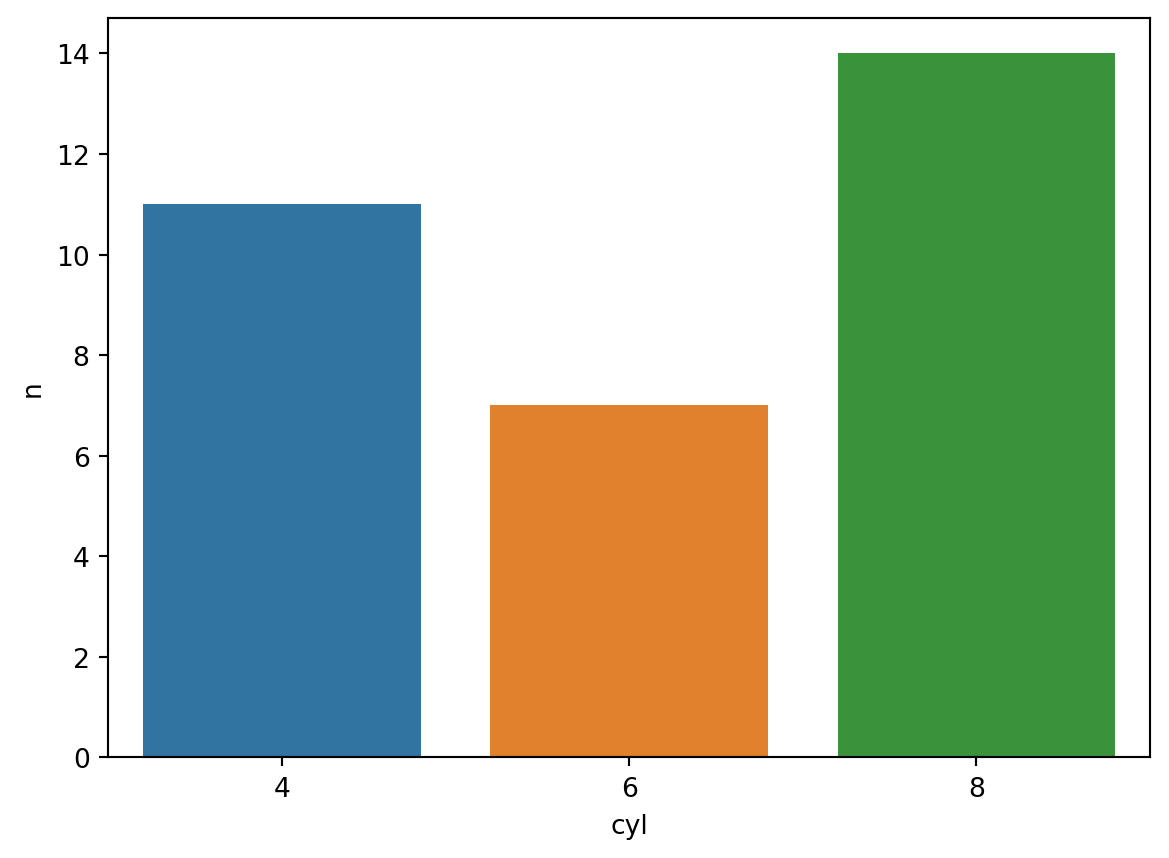A commonly used feature of siuba is the `>>` operator for piping the result of one verb into another. This feature may seem silly compared to method chaining. However, it makes it easy for other packages to contribute new verbs, and lazy pipes can be combined together.

• Piping with the `>>` operator.
• Using `call()` to add external functions to a pipe.
• Using `pipe()` create pipes without `>>`.
``````import pandas as pd

from siuba.siu import _, pipe, call
from siuba import group_by, summarize, inner_join, head, filter
from siuba.data import mtcars``````

## Basic pipe

Use the `>>` operator to pass the result of one verb into another.

``````(mtcars
>> group_by(_.cyl)
>> summarize(res = _.hp.mean())
)``````
cyl res
0 4 82.636364
1 6 122.285714
2 8 209.214286

Note that this is equivalent to the code below, but much more readable.

``````summarize(
group_by(
mtcars,
_.cyl
),
res = _.hp.mean()
)``````
cyl res
0 4 82.636364
1 6 122.285714
2 8 209.214286

## Lazy pipes

Start a pipe with `_` to create a lazy pipes. Lazy pipes are a lot like functions—they run once data is passed to them.

``````filter_cyl = _ >> filter(_.cyl.isin([4, 8]))

mpg cyl disp hp drat wt qsec vs am gear carb
2 22.8 4 108.0 93 3.85 2.32 18.61 1 1 4 1
4 18.7 8 360.0 175 3.15 3.44 17.02 0 0 3 2
6 14.3 8 360.0 245 3.21 3.57 15.84 0 0 3 4
7 24.4 4 146.7 62 3.69 3.19 20.00 1 0 4 2
8 22.8 4 140.8 95 3.92 3.15 22.90 1 0 4 2

Multiple lazy pipes can be combined in a pipe.

``````head_5 = _ >> head(n=5)

mpg cyl disp hp drat wt qsec vs am gear carb
2 22.8 4 108.0 93 3.85 2.32 18.61 1 1 4 1
4 18.7 8 360.0 175 3.15 3.44 17.02 0 0 3 2
6 14.3 8 360.0 245 3.21 3.57 15.84 0 0 3 4
7 24.4 4 146.7 62 3.69 3.19 20.00 1 0 4 2
8 22.8 4 140.8 95 3.92 3.15 22.90 1 0 4 2

This allows them to work as building blocks during an analysis!

## How do verbs work?

The key to using verbs in a pipe is they have two modes, depending on what they receive as their first argument:

• `verb(DataFrame, ...)`: execute the verb right away.
• `verb(...)`: delay execution, and return a `Call`, which can be used in a pipe.

For example, here is a summarize being executed directly.

``summarize(mtcars, avg = _.mpg.mean())``
avg
0 20.090625

Here is an example of a group_by call that could be used in a pipe.

``````group_cyl = group_by(_.cyl)

type(group_cyl)``````
``siuba.siu.calls.Call``
``mtcars >> group_cyl >> summarize(res = _.hp.mean())``
cyl res
0 4 82.636364
1 6 122.285714
2 8 209.214286

### Explicit use of verbs in a pipe

Use `_` as the first argument to a verb, in order to return a `Call`.

``````# eagerly evaluated ----
group_by(mtcars, _.cyl)

# lazy: both of these can be used in a pipe ----

# implicit
group_by(_.cyl)

# explicit
group_by(_, _.cyl)``````
``<function group_by at 0x7f96904954c0>(_,_.cyl())``

This is much more explicit, but also a bit more clunky looking.

## Call two-table verbs

Some verbs take two tables of data. For example, `inner_join()` merges two tables of data based on some condition matching them up.

For two-table verbs, use `_` as the first argument, to indicate it is being used in a pipe.

``````tbl_labels = pd.DataFrame({"cyl": [4, 6, 8], "label": ["four", "six", "eight"]})

# executed right away
inner_join(mtcars, tbl_labels, "cyl")

# piping approach
mtcars >> inner_join(_, tbl_labels, "cyl")``````
mpg cyl disp hp drat wt qsec vs am gear carb label
0 21.0 6 160.0 110 3.90 2.620 16.46 0 1 4 4 six
1 21.0 6 160.0 110 3.90 2.875 17.02 0 1 4 4 six
... ... ... ... ... ... ... ... ... ... ... ... ...
30 15.8 8 351.0 264 4.22 3.170 14.50 0 1 5 4 eight
31 15.0 8 301.0 335 3.54 3.570 14.60 0 1 5 8 eight

32 rows × 12 columns

## Call DataFrame methods

Sometimes it is helpful to use Pandas DataFrame methods, in addition to siuba verbs. This can be done by piping the data to `_.<some_method>()`.

Here is an example using the siuba verb `count()`, with the pandas method `.sort_values()`.

``````from siuba import _, count
from siuba.data import mtcars

(mtcars
>> count(_.cyl)         # this is a siuba verb
>> _.sort_values("n")   # this is a pandas method
)``````
cyl n
1 6 7
0 4 11
2 8 14

Here is another example, using the DataFrame `.shape` attribute.

``````# siuba pipe
mtcars >> _.shape``````
``32``
``````# regular pandas
mtcars.shape``````

## Call external functions

Use `call()` to pipe data into any function call.

The example below pipes to the seaborn’s `barplot` function.

``````from siuba.siu import call
import seaborn as sns

(mtcars
>> count(_.cyl)
>> call(sns.barplot, x="cyl", y="n", data=_)
)``````
``<AxesSubplot: xlabel='cyl', ylabel='n'>``Note that `sns.barplot()` expects the data as a named argument, so we pass `data=_`, where `_` is a placeholder for the data.

`call()` can also take a single function to call the data on.

``````# piping
mtcars >> call(len)``````
``32``
``````# regular function call
len(mtcars)``````
``32``

## `pipe()` as an alternative to `>>`

``````(
mtcars
>> group_by(_.cyl, _.gear)
>> summarize(res = _.hp.mean())
>> call(print, "Printed output -\n", _)
)``````
``````Printed output -
cyl  gear         res
0     4     3   97.000000
1     4     4   76.000000
..  ...   ...         ...
6     8     3  194.166667
7     8     5  299.500000

[8 rows x 3 columns]``````
``````pipe(
mtcars
, group_by(_.cyl, _.gear)
, summarize(res = _.hp.mean())
, call(print, "Printed output -\n", _)
)``````